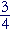# Solving linear equations:  Section 2

Back to Section 1

Canceling

The unknown on both sides

Simple fractional equations

Canceling

If equal terms appear on both sides of an equation,
then we may "cancel" them.

x + b + d  = c + d.

d appears on both sides.  Therefore, we may cancel them.

x + b  = c.

Theoretically, we can say that we subtracted d from both sides.

Finally, on solving for x:

x  = cb.

Problem 19.   Solve for x :

x² + x − 5  =  x² − 3

over the colored area.
Do the problem yourself first!

Cancel the x²'s:

 x − 5 = −3 x = −3 + 5 = 2.

Problem 20.   Solve for x :

xa + b  =  a + b + c

Cancel the b's but not the a's.  On the left is −a, but on the right is +a.  They are not equal.

Transpose −a:

 x = a + a + c x = 2a + c.

The unknown on both sides

Example 4.   Solve for x :

 4x − 3 = 2x − 11. 1.  Transpose the x's to the left and the numbers to the right: 4x − 2x = −11 + 3. 2.  Collect like terms, and solve: 2x = −8 x = −4.

This is another example of doing algebra with your eyes.  In the first line, you should see that 2x goes to the left as −2x, and that −3 goes to the right as +3.

As a general rule for solving any linear equation, we can now state the following:

Transpose all the terms that involve the unknown to the left, and add them;
transpose the remaining terms to the right;
make 1 the final coefficient of the unknown, by dividing or multiplying.

Problem 21.   Solve for x :

 15 + x = 7 + 5x x − 5x = 7 − 15 −4x = −8 x = 2.

Problem 22.   Solve for x :

 1.25x − 6 = x 1.25x −x = 6 (1.25 − 1)x = 6 On combining the like terms on the left. .25 x = 6 x = 24. On multiplying both sides by 4.

Problem 23.   Remove parentheses, add like terms, and solve for x :

 (8x − 2) + (3 − 5x) = (2x − 1) − (x − 3) 8x − 2 + 3 − 5x = 2x − 1 − x + 3 on removing the parentheses 3x + 1 = x + 2 3x − x = 2 − 1 2x = 1 x = 12

Simple fractional equations

 Example 5. x2 =  4.

Since 2 divides on the left, it will multiply on the right:

 x = 2 · 4 = 8.

Problem 24.   Solve for x :

 −x 5 = 3 −x = 15 x = −15.
 Problem 25. x4 = 12 x = 4 · 12 = 2.

Example 6.   Solve for x:

 4x =  5.

Solution.   In the standard form of a simple fractional equation, x is in the numerator.  But we can easily make that standard form by taking the reciprocal of both sides.

 x4 = 15

For if two numbers are equal, then their reciprocals are equal.

 This implies x = 4 · 15
 = 45 .
 Problem 26. 2x = 13 x2 = 3 x = 6.

Example 7.  Fractional coefficient.

 3x4 = y

Since 4 divides on the left, it will multiply on the right:

 3x = 4y.

And since 3 multiplies on the left, it will divide on the right:

 x = 4y 3

In other words,goes to the other side as its reciprocal,.

 Note that 34 is the coefficient of x :
 3x4 = 34 x .

Coefficients go to the other side as their reciprocals.

 Problem 27. 2x3 = a x = 3a 2
 Problem 28. 58 x = a − b x = 85 (a − b)
 Problem 29. 45 x  + 6 = 14 45 x = 14 − 6 = 8 x = 54 · 8 = 10.
 Problem 30. A = ½xB Exchange sides: ½xB = A x = 2A B

The reciprocal of ½ is 2.

Problem 31.   The Celsius temperature C is related to the Fahrenheit temperature F  by this formula,

 F = 95 C + 32
 a) What is the Fahrenheit temperature when the Celsius temperature when the Fahrenheit temperature is 10°?
 F = 95 · 10 + 32 = 18 + 32 = 50°.

b)  Solve the formula for C.

Exchange sides:

 95 C + 32 = F 95 C = F − 32 C = 59 (F − 32).
 c) What is the Celcius temperature when the Fahrenheit temperature when the Fahrenheit temperature is 68°?

20°.

This is Problem 13 of Lesson 1.Back to Section 1

Please make a donation to keep TheMathPage online.
Even \$1 will help.